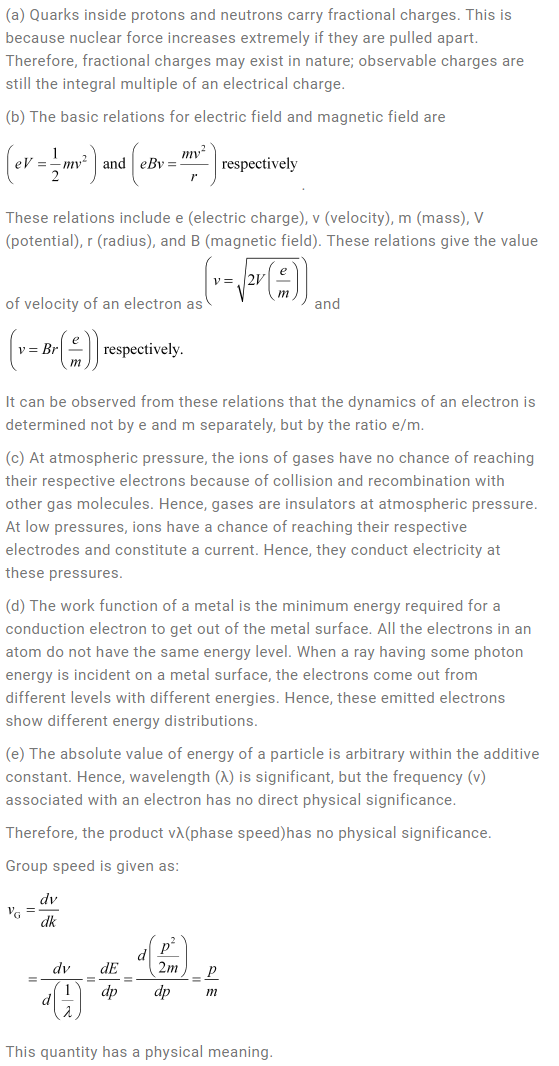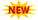SSC Phase 10 Notification || TSSPDCL AE Notification || PPSC Naib Tehsildar Admit Card ||

# NCERT Solutions For Class 12 Physics Chapter 11 Dual Nature of Radiation and Matter

NCERT Solutions For Class 12 Physics Chapter 11 helps us get a better understanding of the discovery of the electron, and their properties, the dual nature of matter with the wide acceptance of Maxwell’s Electromagnetic theory, etc. NCERT Solutions For Class 12 Physics will help students to get their doubts clarified regarding problems in other chapters as well. Dual Nature Of Radiation And Matter Class 12 NCERT Solutions also gives the students to read and know about the discovery of the electron, Cathode ray tube, Photoelectric effect as discovered by Albert Einstein, and its experimental studies etc.

## Class 12 NCERT Physics Chapter 11 Solutions

Subtopics in the NCERT Solutions For Class 12 Physics Chapter 11 are provided in the following table.

 Section Topic Name 11 Dual Nature of Radiation and Matter 11.1 Introduction 11.2 Electron Emission 11.3 Photoelectric Effect 11.4 Experimental Study of Photoelectric Effect 11.5 Photoelectric Effect and Wave Theory of Light 11.6 Einstein’s Photoelectric Equation: Energy Quantum of Radiation 11.7 Particle Nature of Light: The Photon 11.8 Wave Nature of Matter 11.9 Davisson and Germer Experiment

### Dual Nature Of Radiation And Matter Class 12 Physics NCERT Solutions

Question 11.1: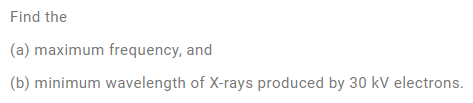Solution: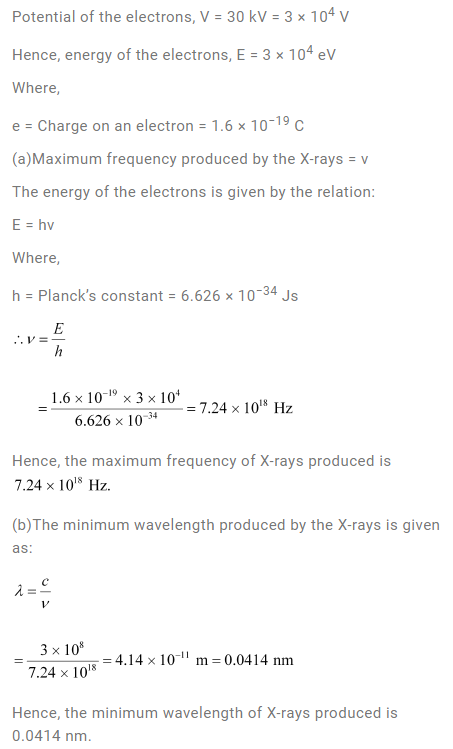Question 11.2:

The work function of caesium metal is 2.14 eV. When light of frequency 6 ×1014 Hz is incident on the metal surface, photoemission of electrons occurs. What is the

(a) maximum kinetic energy of the emitted electrons,

(b) Stopping potential, and

(c) maximum speed of the emitted photoelectrons?

Solution: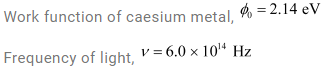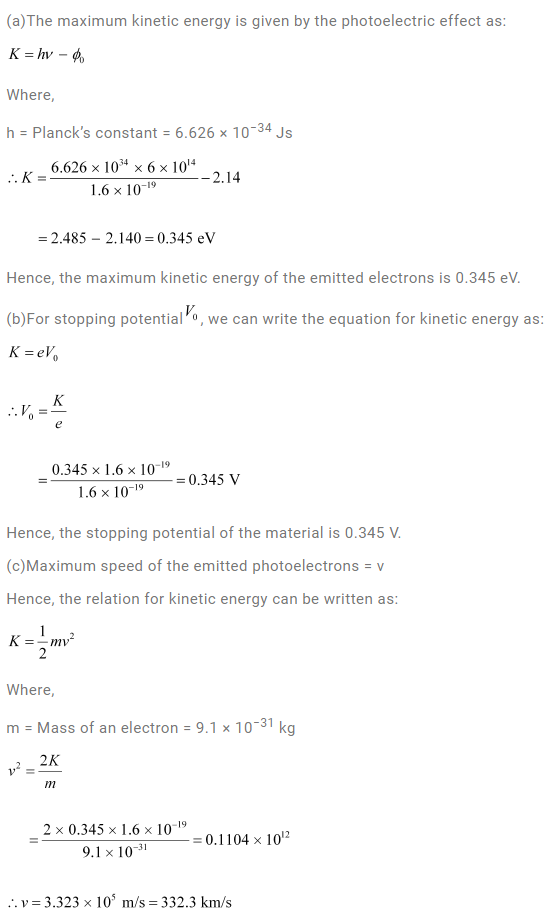Question 11.3:

The photoelectric cut-off voltage in a certain experiment is 1.5 V. What is the maximum kinetic energy of photoelectrons emitted?

Solution: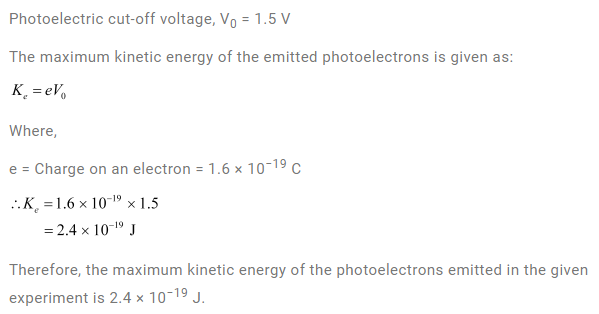Question 11.4:

Monochromatic light of wavelength 632.8 nm is produced by a helium-neon laser. The power emitted is 9.42 mW.

(a) Find the energy and momentum of each photon in the light beam,

(b) How many photons per second, on average, arrive at a target irradiated by this beam? (Assume the beam to have a uniform cross-section which is less than the target area), and

(c) How fast does a hydrogen atom have to travel in order to have the same momentum as that of the photon?

Solution: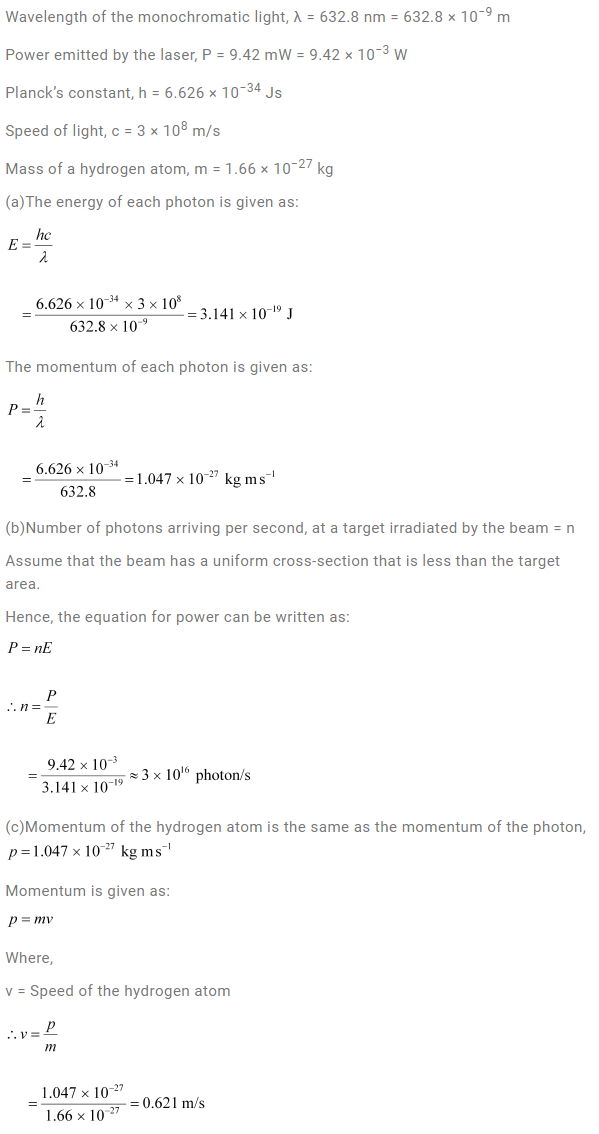Question 11.5:

The energy flux of sunlight reaching the surface of the earth is 1.388 × 103 W/m2. How many photons (nearly) per square metre are incident on the Earth per second? Assume that the photons in the sunlight have an average wavelength of 550 nm.

Solution: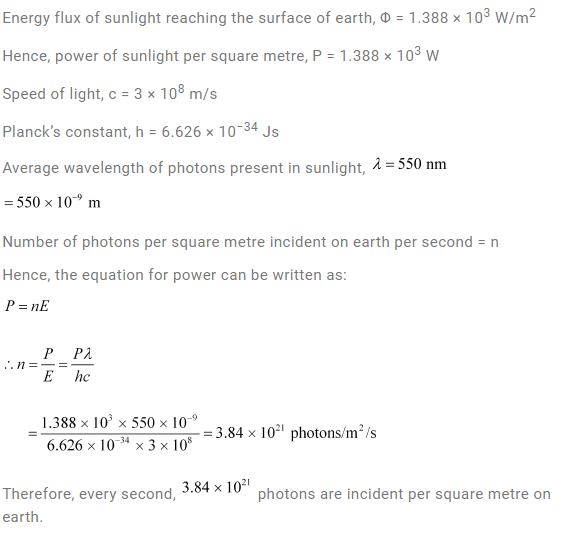Question 11.6:

In an experiment on the photoelectric effect, the slope of the cut-off voltage versus the frequency of incident light is found to be 4.12 × 10−15 V s. Calculate the value of Planck’s constant.

Solution: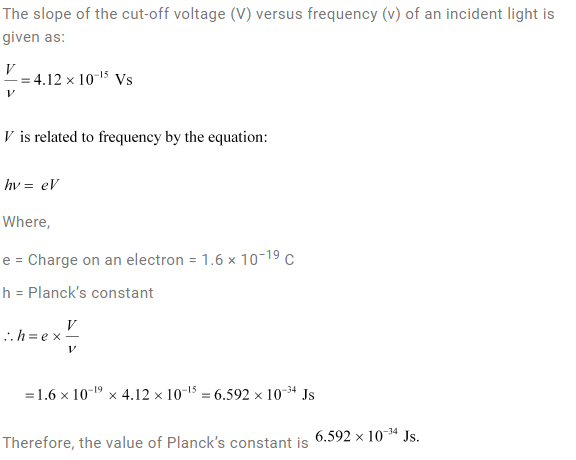Question 11.7:

A 100 W sodium lamp radiates energy uniformly in all directions. The lamp is located at the centre of a large sphere that absorbs all the sodium light which is incident on it. The wavelength of the sodium light is 589 nm. (a) What is the energy per photon associated with the sodium light? (b) At what rate are the photons delivered to the sphere?

Solution: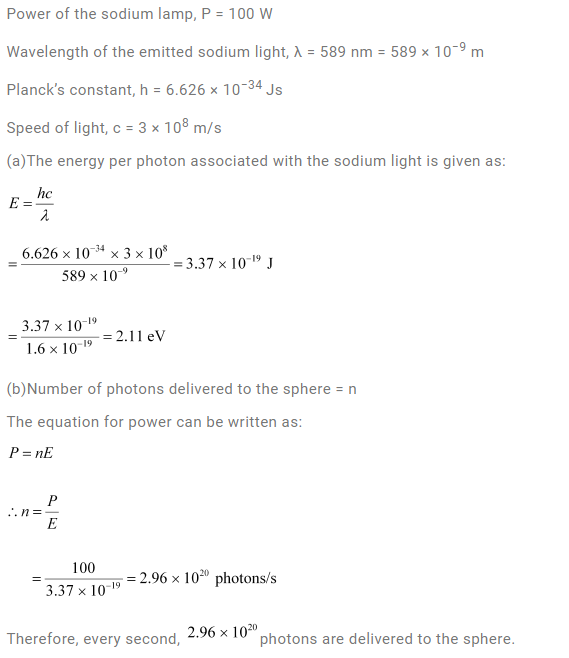Question 11.8:

The threshold frequency for a certain metal is 3.3 × 1014 Hz. If light of frequency 8.2 × 1014 Hz is incident on the metal, predict the cutoff voltage for the photoelectric emission.

Solution: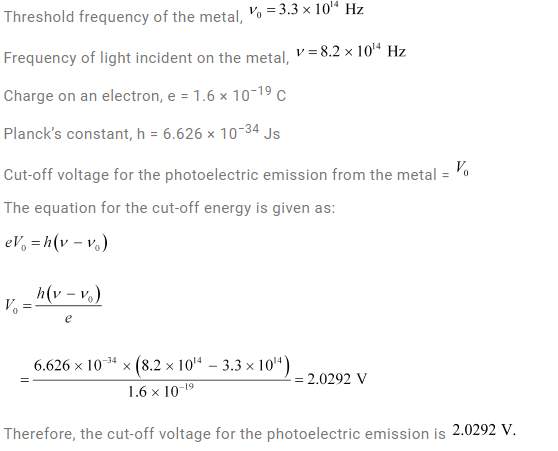Question 11.9:

The work function for a certain metal is 4.2 eV. Will this metal give photoelectric emission for incident radiation of wavelength 330 nm?

Solution: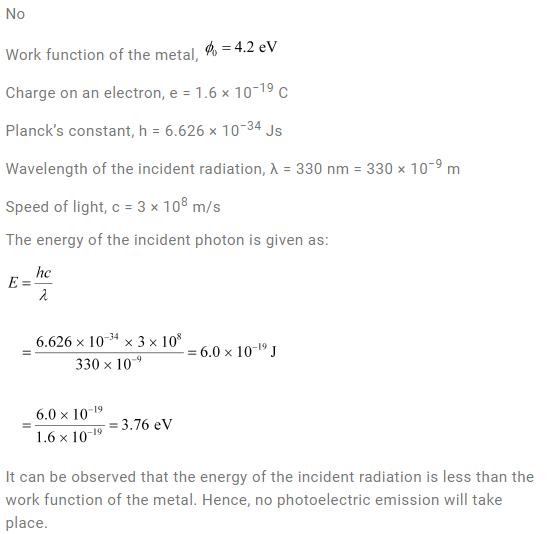Question 11.10:

Light of frequency 7.21 × 1014 Hz is incident on a metal surface. Electrons with a maximum speed of 6.0 × 105 m/s are ejected from the surface. What is the threshold frequency for photoemission of electrons?

Solution: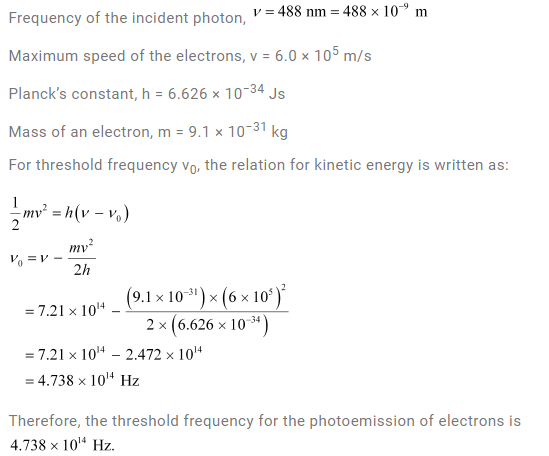Question 11.11:

Light of wavelength 488 nm is produced by an argon laser which is used in the photoelectric effect. When light from this spectral line is incident on the emitter, the stopping (cut-off) potential of photoelectrons is 0.38 V. Find the work function of the material from which the emitter is made.

Solution: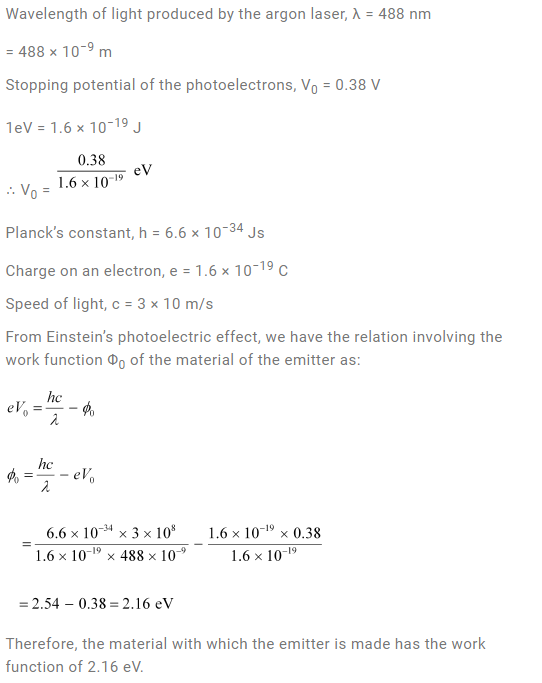Question 11.12:

Calculate the

(a) momentum, and

(b) de Broglie wavelength of the electrons accelerated through a potential difference of 56 V.

Solution: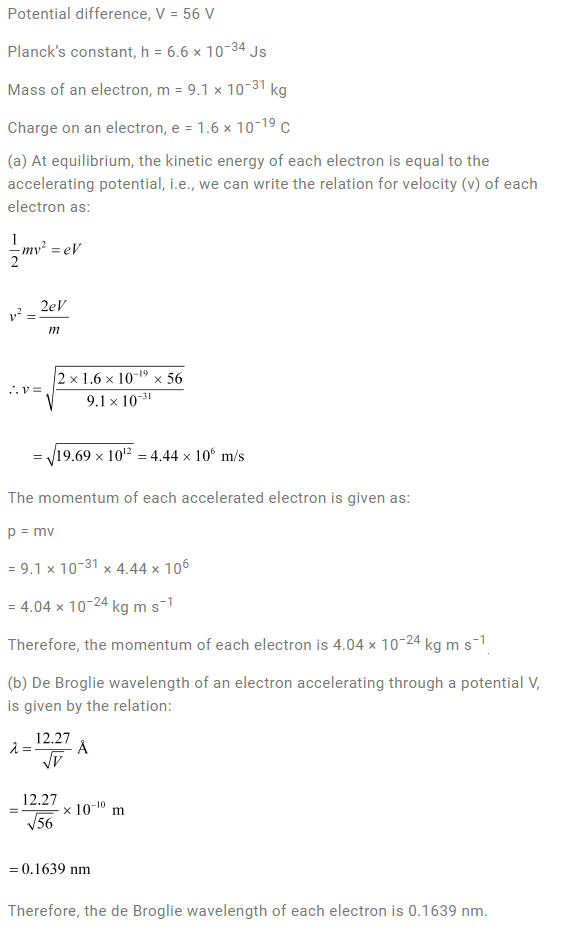Question 11.13:

What is the

(a) momentum,

(b) speed, and

(c) de Broglie wavelength of an electron with kinetic energy of 120 eV.

Solution: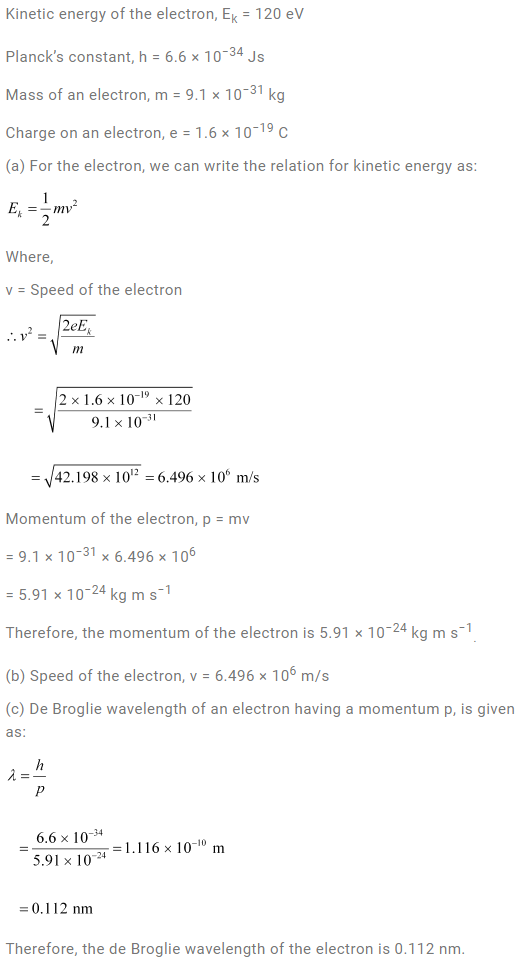Question 11.14:

The wavelength of light from the spectral emission line of sodium is 589 nm. Find the kinetic energy at which

(a) an electron, and

(b) a neutron, would have the same de Broglie wavelength.

Solution: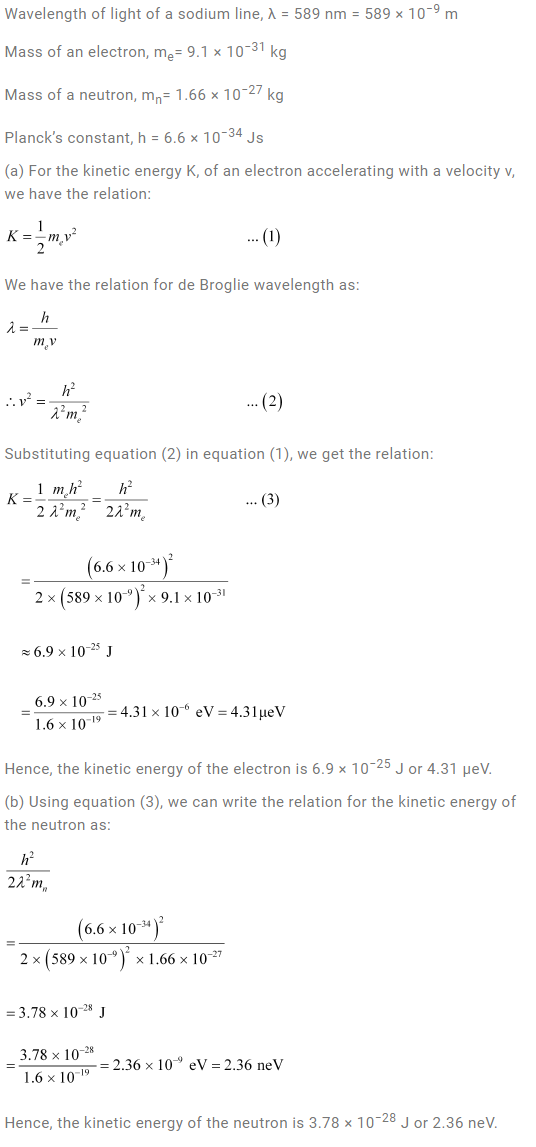Question 11.15:

What is the de Broglie wavelength of

(a) a bullet of mass 0.040 kg travelling at the speed of 1.0 km/s,

(b) a ball of mass 0.060 kg moving at a speed of 1.0 m/s, and

(c) a dust particle of mass 1.0 × 10−9 kg drifting with a speed of 2.2 m/s?

Solution: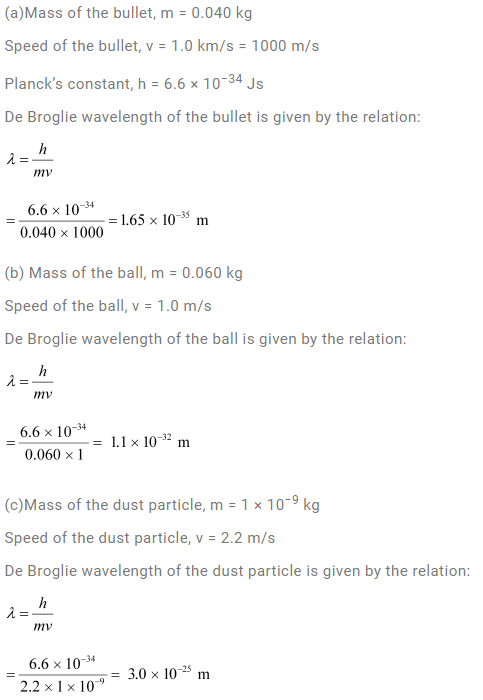Question 11.16:

An electron and a photon each have a wavelength of 1.00 nm. Find

(a) their momenta,

(b) the energy of the photon, and

(c) the kinetic energy of electron.

Solution: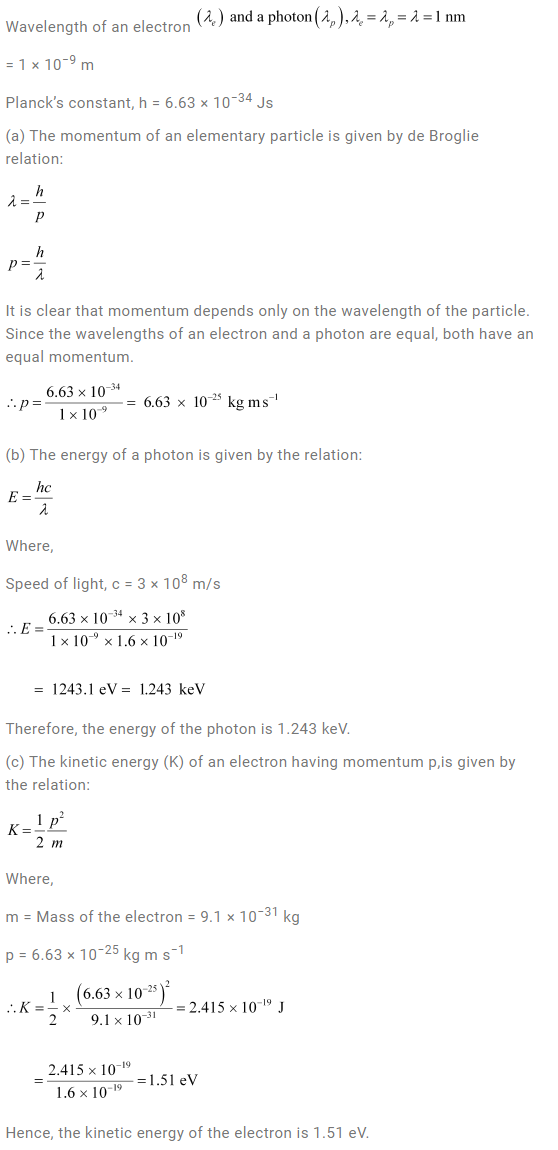Question 11.17:

(a) For what kinetic energy of a neutron will the associated de Broglie wavelength be 1.40 × 10−10 m?

(b) Also find the de Broglie wavelength of a neutron, in thermal equilibrium with matter, having an average kinetic energy of (3/2) kT at 300 K.

Solution: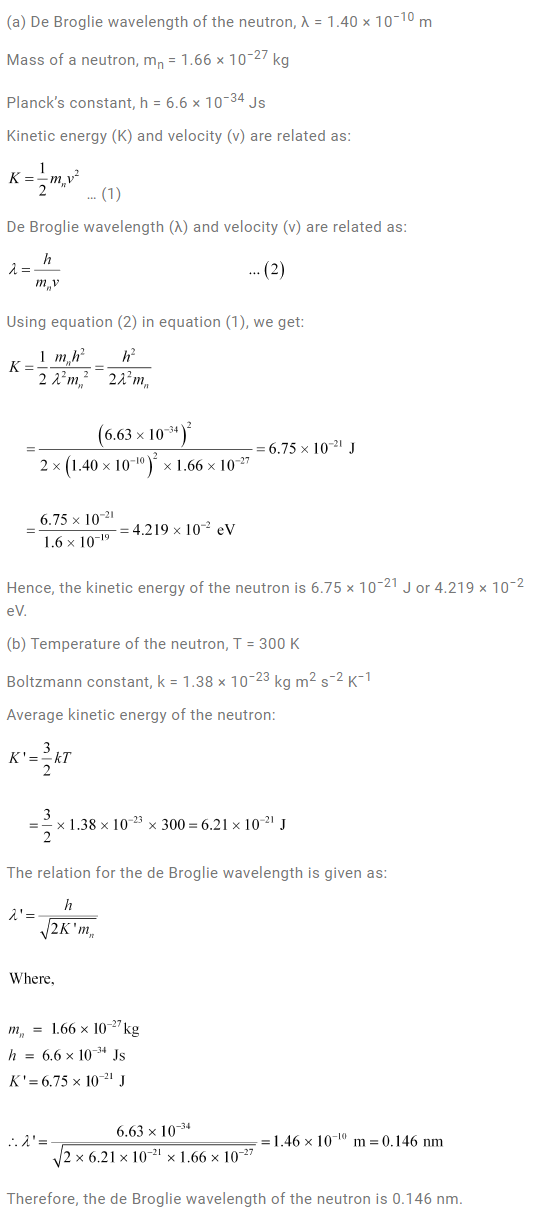Question 11.18:

Show that the wavelength of electromagnetic radiation is equal to the de Broglie wavelength of its quantum (photon).

Solution: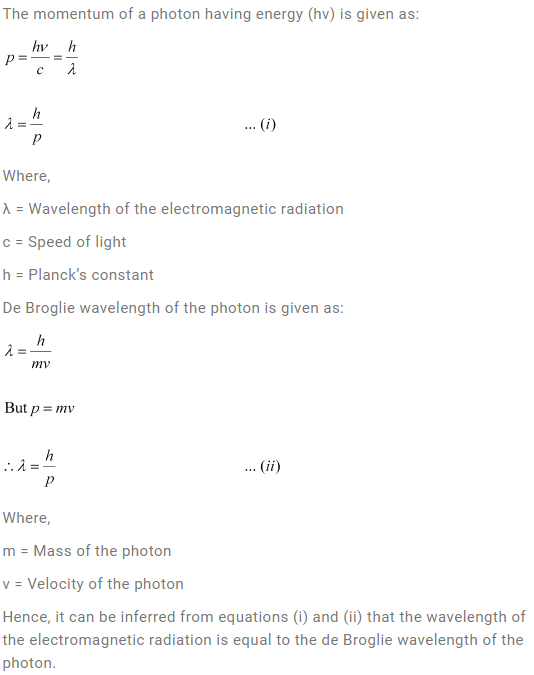Question 11.19:

What is the de Broglie wavelength of a nitrogen molecule in air at 300 K? Assume that the molecule is moving with the root-mean square speed of molecules at this temperature. (Atomic mass of nitrogen = 14.0076 u)

Solution: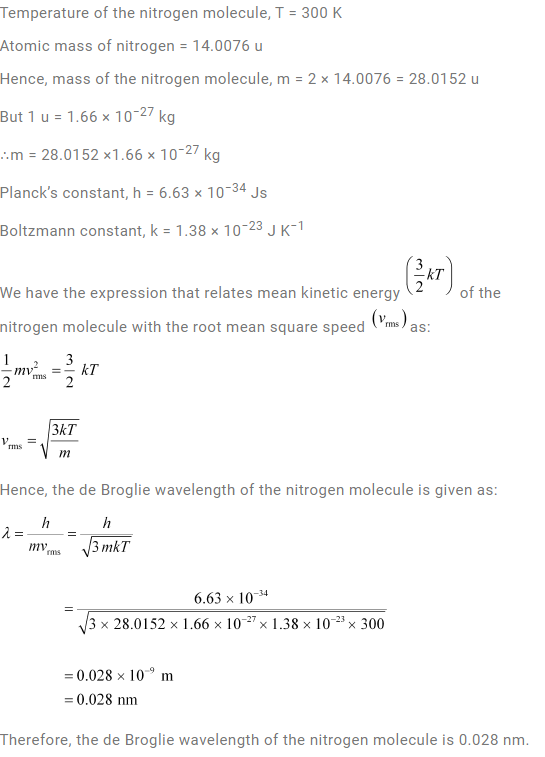Question 11.20:

(a) Estimate the speed with which electrons emitted from a heated emitter of an evacuated tube impinge on the collector maintained at a potential difference of 500 V with respect to the emitter. Ignore the small initial speeds of the electrons. The specific charge of the electron, i.e., its e/m is given to be 1.76 × 1011 C kg−1.

(b) Use the same formula you employ in (a) to obtain electron speed for an collector potential of 10 MV. Do you see what is wrong? In what way is the formula to be modified?

Solution: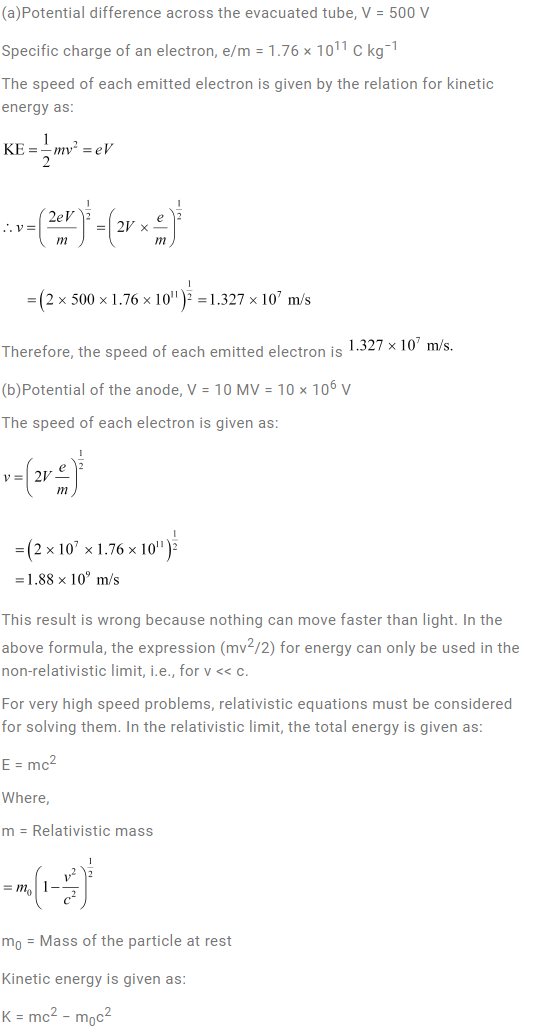Question 11.21:

(a) A monoenergetic electron beam with electron speed of 5.20 × 106 m s−1 is subject to a magnetic field of 1.30 × 10−4 T normal to the beam velocity. What is the radius of the circle traced by the beam, given e/m for electron equals 1.76 × 1011 C kg−1.

(b) Is the formula you employ in (a) valid for calculating radius of the path of a 20 MeV electron beam? If not, in what way is it modified?

[Note: Exercises 11.20(b) and 11.21(b) take you to relativistic mechanics which is beyond the scope of this book. They have been inserted here simply to emphasize the point that the formulas you use in part (a) of the exercises are not valid at very high speeds or energies. See answers at the end to know what ‘very high speed or energy’ means.]

Solution: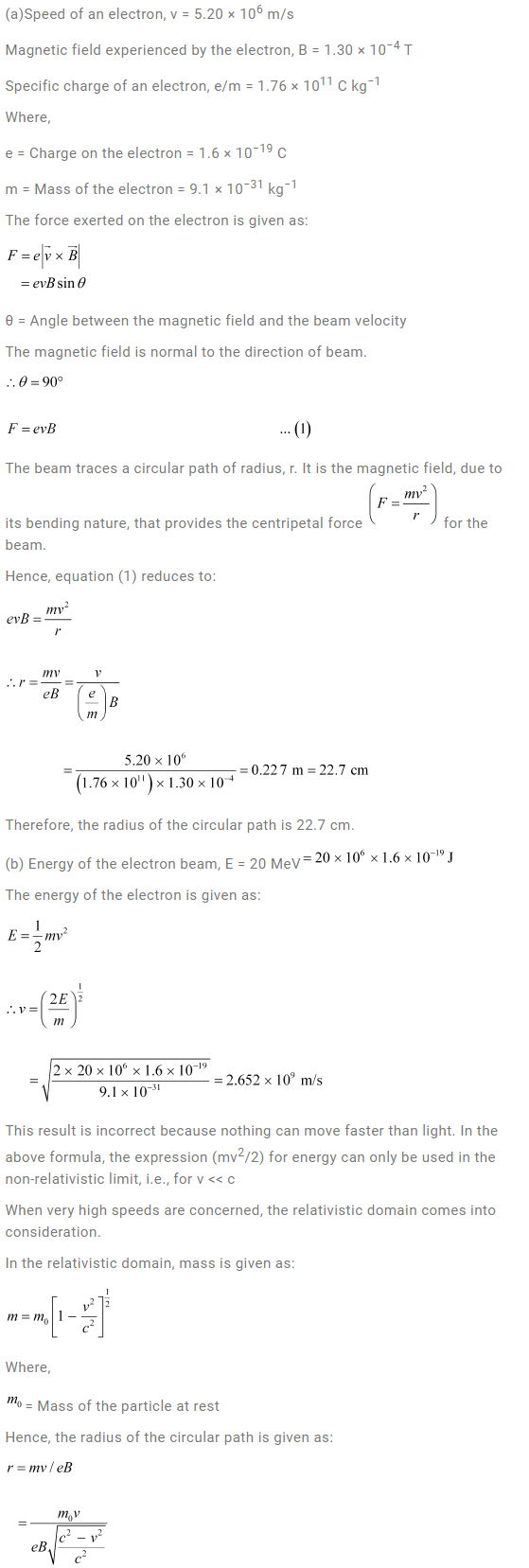Question 11.22:

An electron gun with its collector at a potential of 100 V fires out electrons in a spherical bulb containing hydrogen gas at low pressure (∼10−2 mm of Hg). A magnetic field of 2.83 × 10−4 T curves the path of the electrons in a circular orbit of radius 12.0 cm. (The path can be viewed because the gas ions in the path focus the beam by attracting electrons, and emitting light by electron capture; this method is known as the ‘fine beam tube’ method. Determine e/m from the data.

Solution: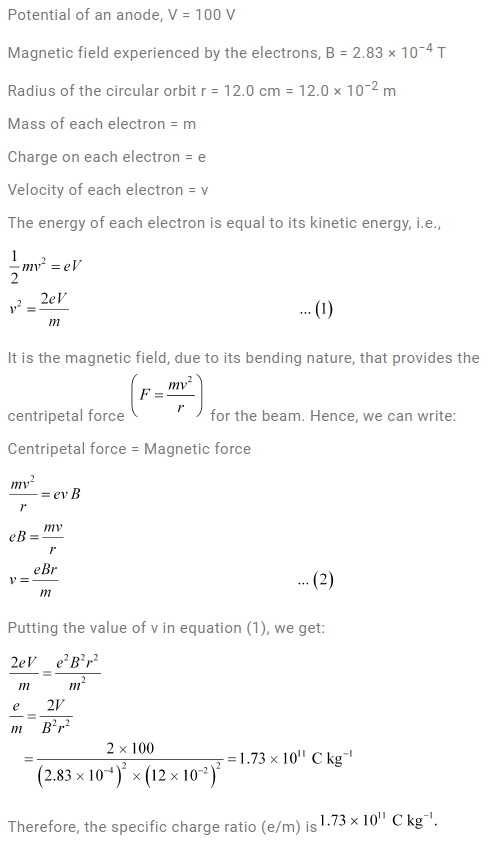Question 11.23:

(a) An X-ray tube produces a continuous spectrum of radiation with its short wavelength end at 0.45 Å. What is the maximum energy of a photon in the radiation?

(b) From your answer to (a), guess what order of accelerating voltage (for electrons) is required in such a tube?

Solution: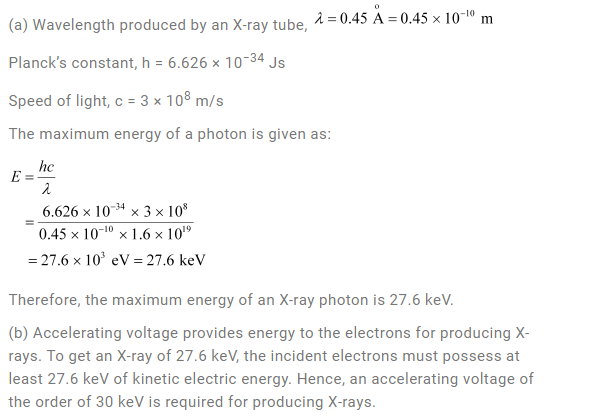Question 11.24:

In an accelerator experiment on high-energy collisions of electrons with positrons, a certain event is interpreted as annihilation of an electron-positron pair of total energy 10.2 BeV into two γ-rays of equal energy. What is the wavelength associated with each γ-ray? (1BeV = 109 eV)

Solution: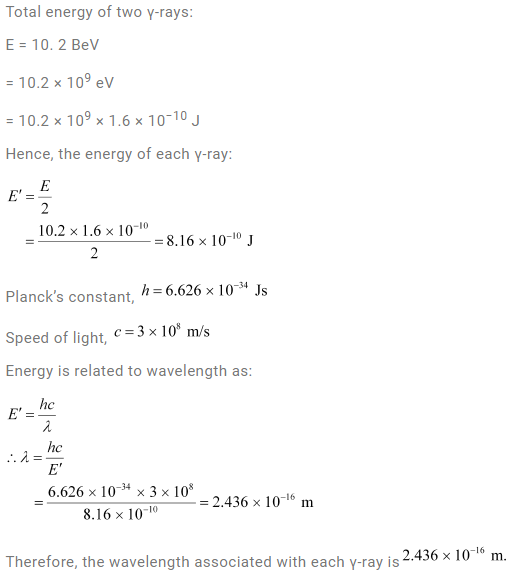Question 11.25:

Estimating the following two numbers should be interesting. The first number will tell you why radio engineers do not need to worry much about photons! The second number tells you why our eye can never ‘count photons’, even in barely detectable light.

(a) The number of photons emitted per second by a Medium wave transmitter of 10 kW power, emitting radio waves of wavelength 500 m.

(b) The number of photons entering the pupil of our eye per second corresponding to the minimum intensity of white light that we humans can perceive (∼10−10 W m−2). Take the area of the pupil to be about 0.4 cm2, and the average frequency of white light to be about 6 × 1014 Hz.

Solution: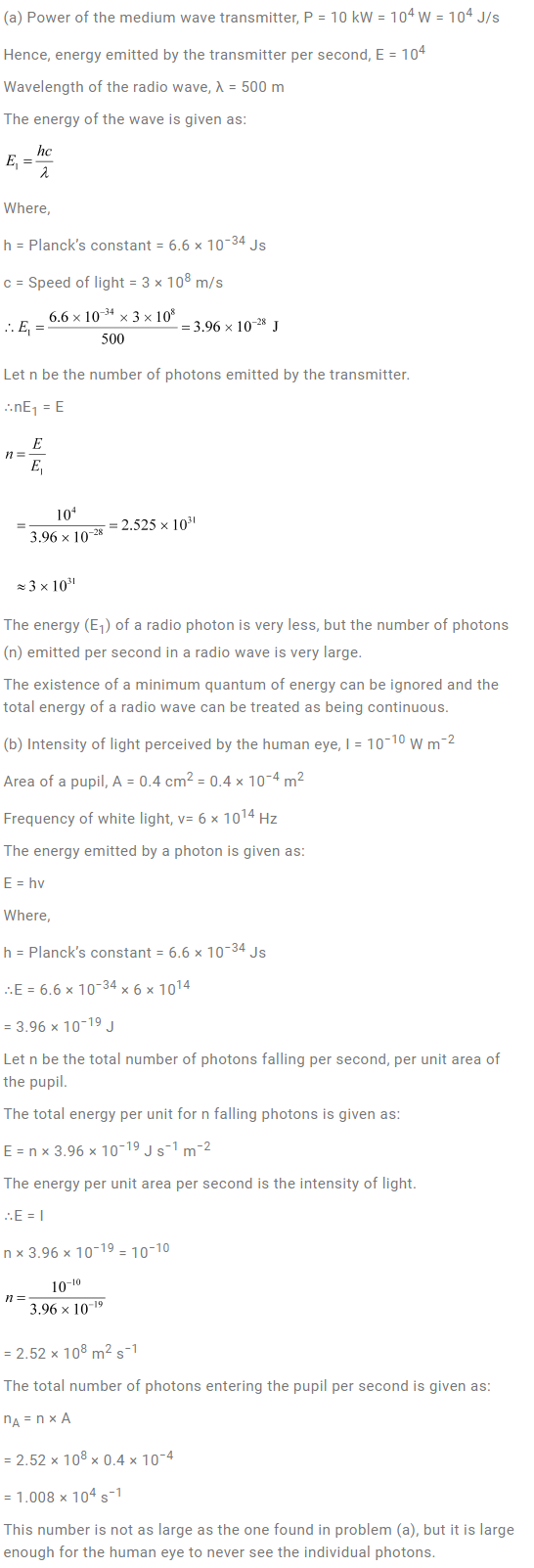Question 11.26

Ultraviolet light of wavelength 2271 Å from a 100 W mercury source irradiates a photo-cell made of molybdenum metal. If the stopping potential is −1.3 V, estimate the work function of the metal. How would the photo-cell respond to a high intensity (∼105 W m−2) red light of wavelength 6328 Å produced by a He-Ne laser?

Solution: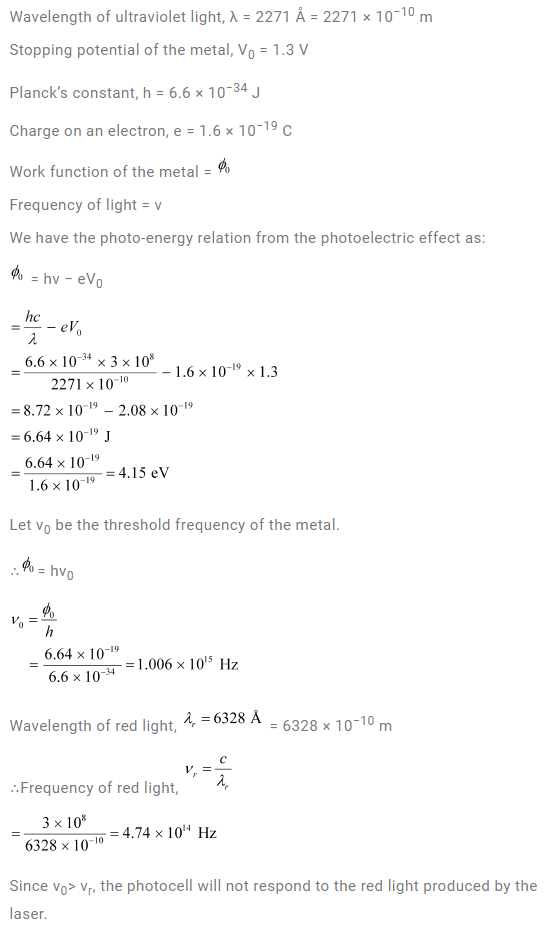Question 11.27:

Monochromatic radiation of wavelength 640.2 nm (1nm = 10−9 m) from a neon lamp irradiates photosensitive material made of Caesium on tungsten. The stopping voltage is measured to be 0.54 V. The source is replaced by an iron source and its 427.2 nm line irradiates the same photo-cell. Predict the new stopping voltage.

Solution: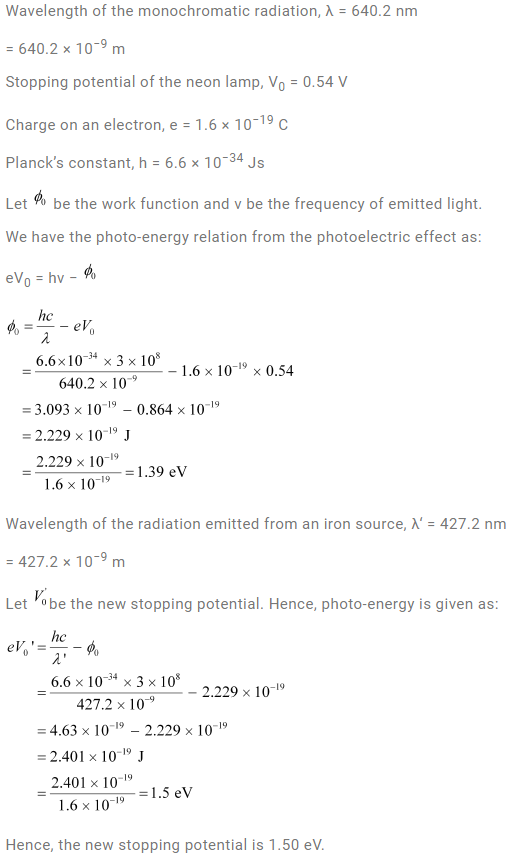Question 11.28:

A mercury lamp is a convenient source for studying frequency dependence of photoelectric emission, since it gives a number of spectral lines ranging from the UV to the red end of the visible spectrum. In our experiment with rubidium photo-cell, the following lines from a mercury source were used:

λ1 = 3650 Å, λ2= 4047 Å, λ3= 4358 Å, λ4= 5461 Å, λ5= 6907 Å,

The stopping voltages, respectively, were measured to be:

V01 = 1.28 V, V02 = 0.95 V, V03 = 0.74 V, V04 = 0.16 V, V05 = 0 V

Determine the value of Planck’s constant h, the threshold frequency and work function for the material.

[Note: You will notice that to get from the data, you will need to know (which you can take to be 1.6 × 10−19 C). Experiments of this kind on Na, Li, K, etc. were performed by Millikan, who, using his own value of (from the oil-drop experiment) confirmed Einstein’s photoelectric equation and at the same time gave an independent estimate of the value of h.]

Solution: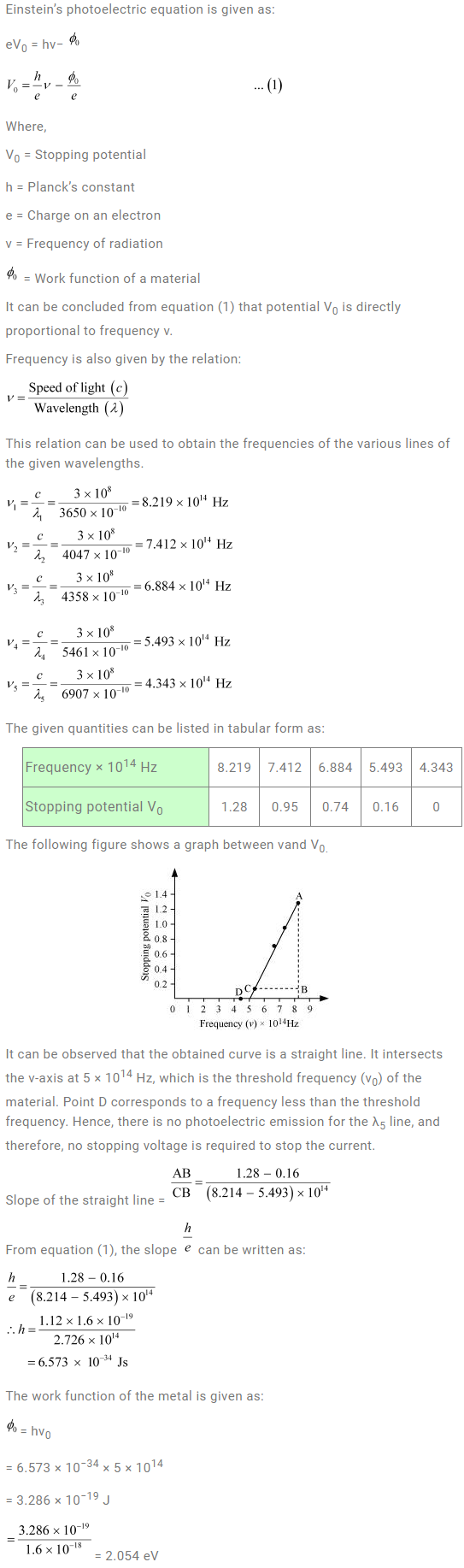Question 11.29:

The work function for the following metals is given:

Na: 2.75 eV; K: 2.30 eV; Mo: 4.17 eV; Ni: 5.15 eV. Which of these metals will not give photoelectric emission for a radiation of wavelength 3300 Å from a He-Cd laser placed 1 m away from the photocell? What happens if the laser is brought nearer and placed 50 cm away?

Solution: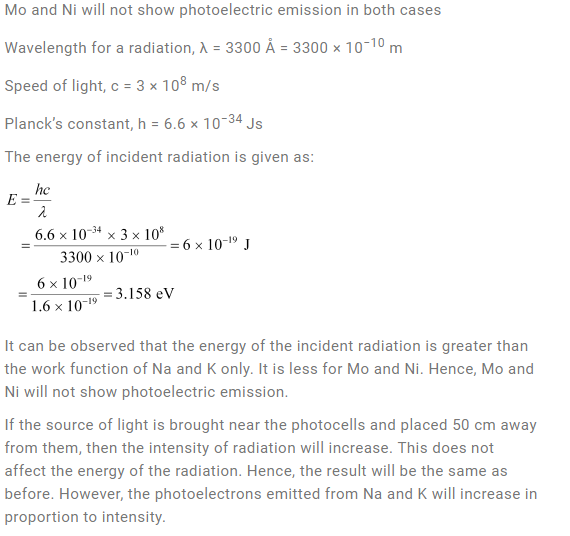Question 11.30:

Light of intensity 10−5 W m−2 falls on a sodium photo-cell of surface area 2 cm2. Assuming that the top 5 layers of sodium absorb the incident energy, estimate time required for photoelectric emission in the wave-picture of radiation. The work function for the metal is given to be about 2 eV. What is the implication of your answer?

Solution: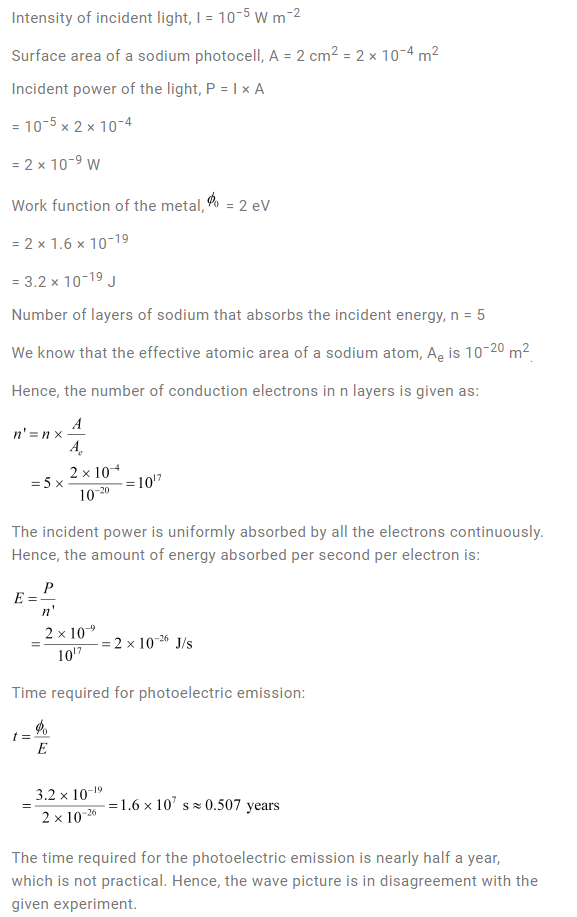Question 11.31:

Crystal diffraction experiments can be performed using X-rays, or electrons accelerated through appropriate voltage. Which probe has greater energy? (For quantitative comparison, take the wavelength of the probe equal to 1 Å, which is of the order of inter-atomic spacing in the lattice) (me= 9.11 × 10−31 kg).

Solution: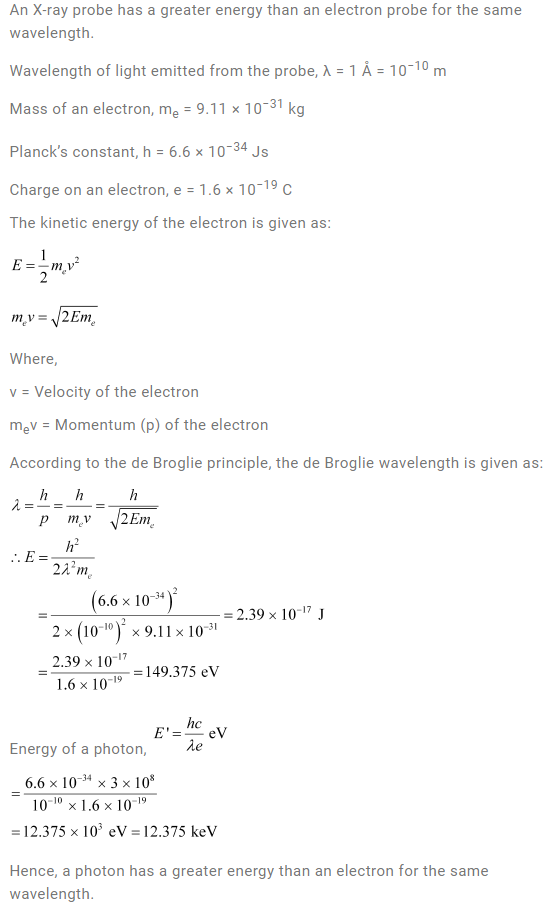Question 11.32:

(a) Obtain the de Broglie wavelength of a neutron of kinetic energy 150 eV. As you have seen in Exercise 11.31, an electron beam of this energy is suitable for crystal diffraction experiments. Would a neutron beam of the same energy be equally suitable? Explain. (mn= 1.675 × 10−27 kg)

(b) Obtain the de Broglie wavelength associated with thermal neutrons at room temperature (27 ºC). Hence explain why a fast neutron beam needs to be thermalized with the environment before it can be used for neutron diffraction experiments.

Solution: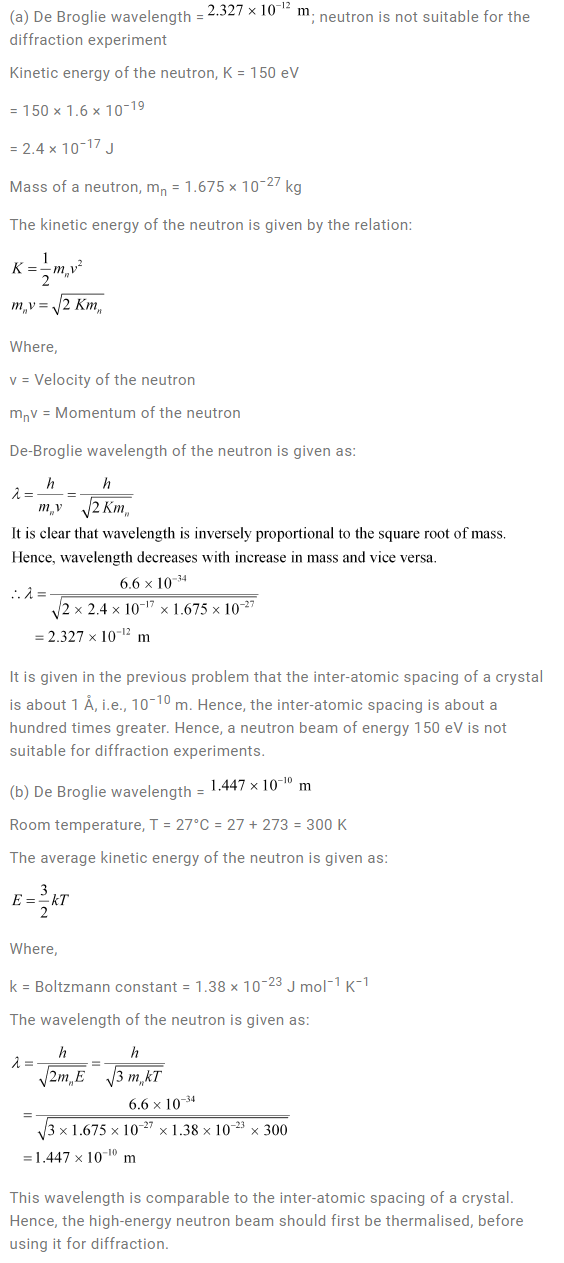Question 11.33:

An electron microscope uses electrons accelerated by a voltage of 50 kV. Determine the de Broglie wavelength associated with the electrons. If other factors (such as numerical aperture, etc.) are taken to be roughly the same, how does the resolving power of an electron microscope compare with that of an optical microscope which uses yellow light?

Solution: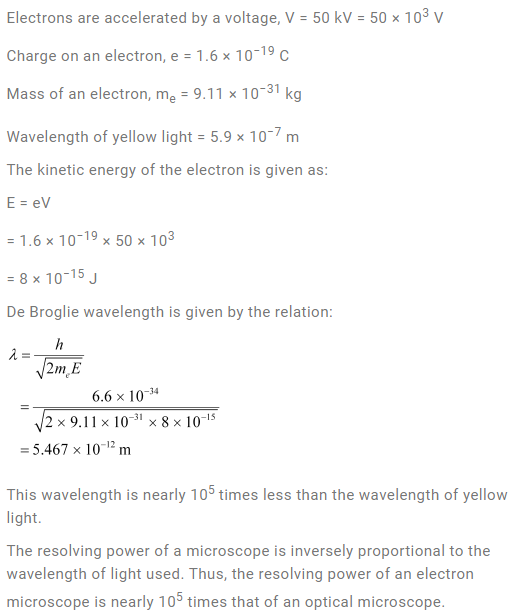Question 11.34:

The wavelength of a probe is roughly a measure of the size of a structure that it can probe in some detail. The quark structure of protons and neutrons appears at the minute length-scale of 10−15 m or less. This structure was first probed in early 1970’s using high energy electron beams produced by a linear accelerator at Stanford, USA. Guess what might have been the order of energy of these electron beams. (Rest mass energy of electron = 0.511 MeV.)

Solution: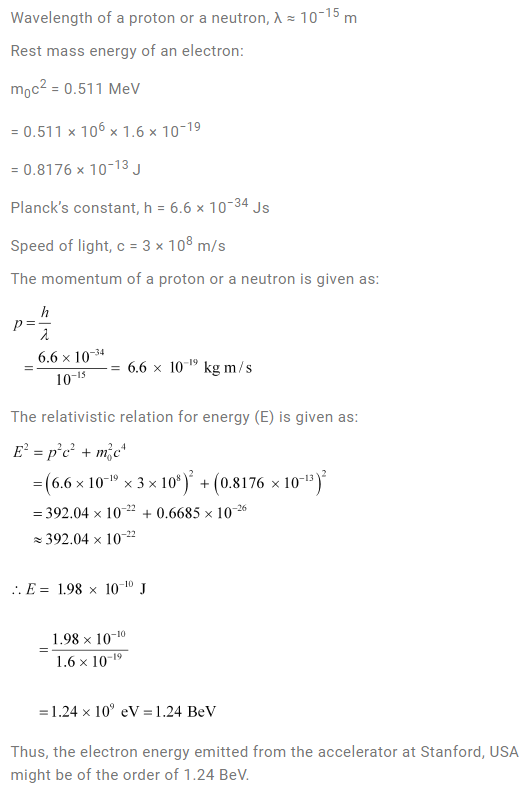Question 11.35:

Find the typical de Broglie wavelength associated with a He atom in helium gas at room temperature (27 ºC) and 1 atm pressure; and compare it with the mean separation between two atoms under these conditions.

Solution: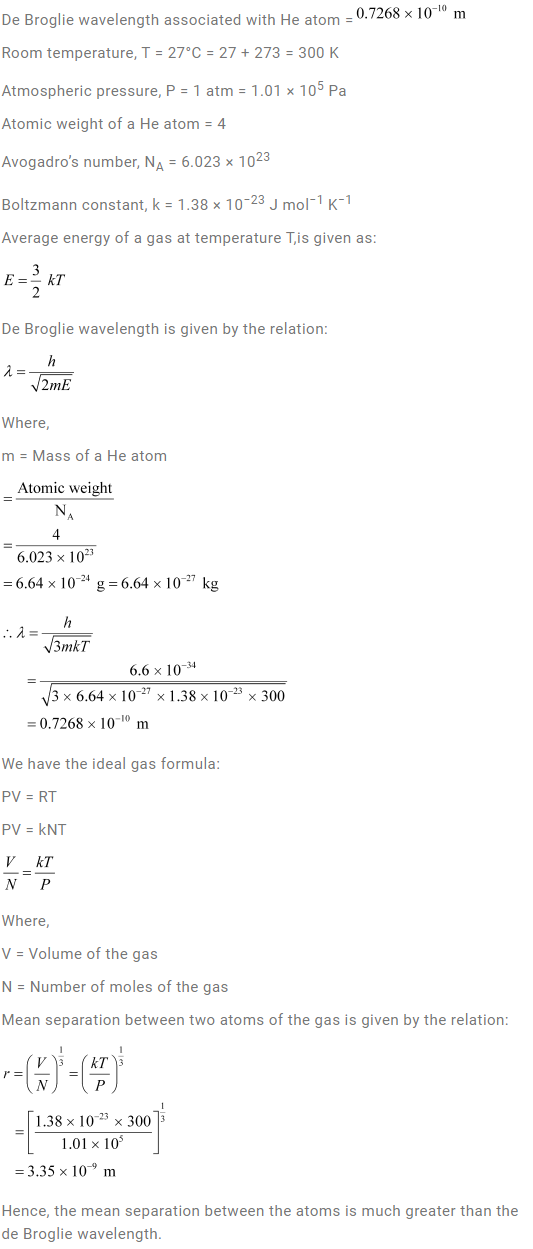Question 11.36:

Compute the typical de Broglie wavelength of an electron in a metal at 27 ºC and compare it with the mean separation between two electrons in a metal which is given to be about 2 × 10−10 m.

[Note: Exercises 11.35 and 11.36 reveal that while the wave-packets associated with gaseous molecules under ordinary conditions are non-overlapping, the electron wave-packets in a metal strongly overlap with one another. This suggests that whereas molecules in an ordinary gas can be distinguished apart, electrons in a metal cannot be distinguished apart from one another. This indistinguishability has many fundamental implications which you will explore in more advanced Physics courses.]

Solution: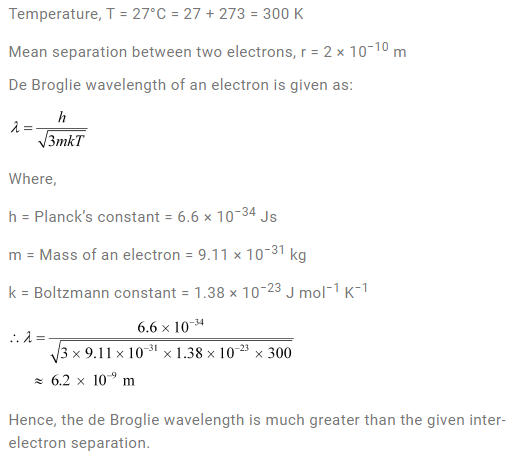Question 11.37:

(a) Quarks inside protons and neutrons are thought to carry fractional charges [(+2/3); (−1/3)e]. Why do they not show up in Millikan’s oil-drop experiment?

(b) What is so special about the combination e/m? Why do we not simply talk of and separately?

(c) Why should gases be insulators at ordinary pressures and start conducting at very low pressures?

(d) Every metal has a definite work function. Why do all photoelectrons not come out with the same energy if incident radiation is monochromatic? Why is there an energy distribution of photoelectrons?

(e) The energy and momentum of an electron are related to the frequency and wavelength of the associated matter wave by the relations:

E = hν= h / λ

But while the value of λ is physically significant, the value of ν (and therefore, the value of the phase speed νλ) has no physical significance. Why?

Solution: